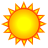# If/Then statement in Model Builder

1900
1
08-01-2012 12:18 PMNew Contributor
I am new to using VBA/ Python and am trying to write an if/then code. In model builder, I am trying to write an If/Then statement for a newly created field. I want to populate the newly added field by stating the following in the Calculate Field tool:

For this new field
If the value of an existing field is greater than 75
Then the value for this new field should be 1
If the value of an existing field is less than 75
Then the value for this new field should be 0

Also, I have added another field and I would like the field to be populated like this:

If the value of the existing field is 1, take the value from the existing field
If the value of the existing field is 0, take the value from the existing field

I have searched the forums and cannot find anything to help me complete this task. Thank you in advance for any help or support you can provide.
Tags (3)byEsri Frequent Contributor
The Calculate Field Examples help article should give you some ideas.

This example comes from that page:

```Expression:
density

Expression Type:
VB

Code Block:
Dim density
If [POP90_SQMI] < 100 Then
density = "low"

elseif [POP90_SQMI] < 300 Then
density = "medium"

else
density = "high"
end if```